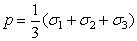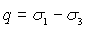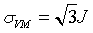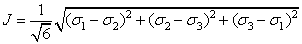The Rocscience International Conference 2021 Proceedings are now available. Read Now

# Principal Stress

The following principal stress data can be contoured.

## Sigma 1

The Sigma 1 option will plot contours of the MAJOR IN-PLANE PRINCIPAL STRESS.

Whenever a file is opened, or when a new window is opened for an already open file, the default plot will always be a Sigma 1 contour plot.

Remember that the in-plane Sigma 1 may not always be the major principal stress in 3-dimensions – if the value of Sigma Z is greater than Sigma 1 at a given point, then the in-plane Sigma 1 will actually be the 3-d intermediate principal stress.

## Sigma 3

The Sigma 3 option will plot contours of the MINOR IN-PLANE PRINCIPAL STRESS.

Remember that the in-plane Sigma 3 may not always be the minor principal stress in 3-dimensions – if the value of Sigma Z is less than Sigma 3 at a given point, then the in-plane Sigma 3 will actually be the 3-d intermediate principal stress

## Sigma Z

The Sigma Z option will plot contours of the OUT-OF-PLANE PRINCIPAL STRESS.

Remember that Sigma Z is not necessarily the intermediate principal stress – depending on the in-plane values of Sigma 1 and Sigma 3 at a given point, Sigma Z could be the 3-dimensional major, intermediate or minor principal stress.

Axisymmetric Sigma Z

If you are analyzing an Axisymmetric problem, then Sigma Z will represent the circumferential stress around the excavation.

## Mean Stress

The Mean Stress (p) is given by:The major, intermediate and minor principal stress correspond to Sigma 1, Sigma Z and Sigma 3 in RS2, but note that Sigma Z can be the major, intermediate or minor principal stress, depending on the magnitudes of Sigma 1 and Sigma 3.

## Differential Stress

The Differential Stress is given by:where Sigma 1 and Sigma 3 are the three-dimensional major and minor principal stress, not necessarily the in-plane principal stresses.

## Von Mises Stress

The Von Mises Stress is given by:where J is given by:The major, intermediate and minor principal stress correspond to Sigma 1, Sigma Z and Sigma 3 in RS2, but note that Sigma Z can be the major, intermediate or minor principal stress, depending on the magnitudes of Sigma 1 and Sigma 3.